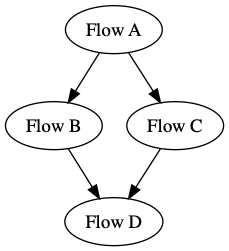# Running dependent flows

There are many situations where users want to configure dependencies at the flow level; for example, if you have a data preprocessing flow that you maintain separately from a model training flow, and you always want to ensure that the preprocessing flow runs before the training flow.

To support this, Prefect provides a convenient built-in task for creating Flow runs through the Prefect API. This task supports:

• triggering flow runs when certain conditions are met in another flow
• providing parameter values that are generated at runtime by another flow
• constructing a Flow-of-Flows that orchestrates and schedules groups of flows simultaneously
• always triggering the most recent version of a flow (so IDs / versions are not hardcoded)
• optionally mirroring the state of the flow run in the task itself
• stable retries to prevent duplication of runs

# Running a parametrized flow

Let's say that you want to always run a flow with parameters that are generated by another flow. Naively, you might manually wait for one flow to finish and then manually trigger the next flow to run with the appropriate parameter values. Prefect makes this pattern easy to automate via the create_flow_run and wait_for_flow_run:

def extract_some_data():
return "some-random-piece-of-data"

with Flow("parent-flow") as flow:
data = extract_some_data()
# assumes you have registered a flow named "example" in a project named "examples"
flow_run = create_flow_run(flow_name="example",
project_name="examples",
parameters={"param-key": data})

# Scheduling a Flow-of-Flows

Oftentimes different people are responsible for maintaining different flows; in this case it can be useful to construct a Flow-of-Flows that specifies execution order dependencies between various Flows.
The wait_for_flow_run task allows you to specify that the task should wait until the triggered flow run completes and reflect the flow run state as the task state.

The following example creates the following Flow-of-Flows that runs every weekday:from prefect import Flow
from prefect.schedules import CronSchedule

weekday_schedule = CronSchedule(
"30 9 * * 1-5", start_date=pendulum.now(tz="US/Eastern")
)

with Flow("parent-flow", schedule=weekday_schedule) as flow:

# assumes you have registered the following flows in a project named "examples"
flow_a = create_flow_run(flow_name="A", project_name="examples")
wait_for_flow_a = wait_for_flow_run(flow_a, raise_final_state=True)

flow_b = create_flow_run(flow_name="B", project_name="examples")
wait_for_flow_b = wait_for_flow_run(flow_b, raise_final_state=True)

flow_c = create_flow_run(flow_name="C", project_name="examples")
wait_for_flow_c = wait_for_flow_run(flow_c, raise_final_state=True)

flow_d = create_flow_run(flow_name="D", project_name="examples")
wait_for_flow_d = wait_for_flow_run(flow_d, raise_final_state=True)

flow_b.set_upstream(wait_for_flow_a)
flow_c.set_upstream(wait_for_flow_a)
flow_d.set_upstream(wait_for_flow_b)
flow_d.set_upstream(wait_for_flow_c)